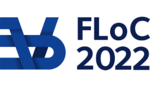FLOC 2022: FEDERATED LOGIC CONFERENCE 2022
TALK KEYWORD INDEX

A
ACL2(r)
algebraic hierarchy
algebraic number theory
algebraic structures
automated theorem proving
automatic test-case reduction
Automatic theorem proving
B
back-and-forth method
Banach-Tarski
bug minimizer
C
canonical structures
Cauchy integral formula
Cauchy-Goursat theorem
certificate checking
choreography
class field theory
Combinatorics
compilation
Compiler verification
Completeness
complex analysis
Compositional Verification
computability theory
computable isomorphisms
concentration inequalities
concurrency
Concurrent Separation Logic
constructive matemathics
constructive type theory
Coq
cryptography
cyclicity
D
debugging
Deep Embedding
definitions
dependent type theory
diophantine sets
divergence theorem
DPRM theorem
E
EasyCrypt
elementary functions
Elpi
Events
F
finite models
first-order logic
formal mathematics
formal proof
Formal Proof Techniques
formal verification
formalization
formalization of mathematics
Frequency Moments
functional analysis
G
Gauge integral
graph theory
Green's theorem
H
Higher-order logic
Hilbert space
hoare logic
HOL Light
HOL4
I
Interactive Theorem Proving
ISA specification
Isabelle
Isabelle Sledgehammer
Isabelle/HOL
K
Kolmogorov complexity
L
lambda calculus
Lean
Lean prover
Linear Algebra
LLVM
lower bound
M
machine learning
Mathematical Components
Mathematical Formalisation
Mathematics
mathlib
measure theory
Mizar
model checking
models of computation
N
Neuronal networks
non-termination
O
Optimization Algorithms
P
packed classes
Parallel Sorting Algorithms
polynomial approximation
Premise Selection
Probability Theory
Program Verification
projective geometry
Proof assistants
Proof automation
proof by reflection
Prover
Q
query model
R
random numbers
Randomized Algorithms
Reduction strategies
Refinement
reinforcement learning
Remez algorithm
rewriting engines
Rotations
Roth's Theorem
S
SeCaV
semilinear
shallow embedding
soundness
statistics
Stochastic Approximation
Stochastic Processes
synthetic computability
synthetic computability theory
Szemerédi's Regularity Lemma
T
Theorem prover soundness
theorem proving
type classes
U
Undecidability
upper bound
User interface
λ
λProlog